### Nonlinear Systems of Equations: Newton-Raphson Method

### Newton-Raphson Method

The Newton-Raphson method is the method of choice for solving nonlinear systems of equations. Many engineering software packages (especially finite element analysis software) that solve nonlinear systems of equations use the Newton-Raphson method. The derivation of the method for nonlinear systems is very similar to the one-dimensional version in the root finding section. Assume a nonlinear system of equations of the form: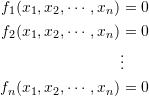If the components of one iteration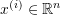are known as: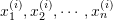, then, the Taylor expansion of the first equation around these components is given by: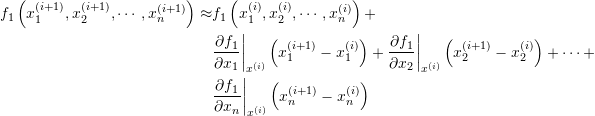Applying the Taylor expansion in the same manner for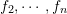, we obtained the following system of linear equations with the unknowns being the components of the vector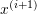:By setting the left hand side to zero (which is the desired value for the functions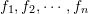, then, the system can be written as: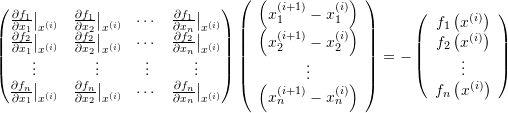Setting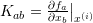, the above equation can be written in matrix form as follows: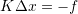where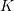is an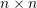matrix,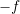is a vector ofcomponents and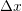is an-dimensional vector with the components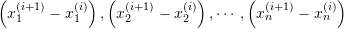. Ifis invertible, then, the above system can be solved as follows: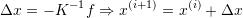#### Example

Use the Newton-Raphson method with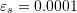to find the solution to the following nonlinear system of equations: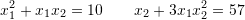##### Solution

In addition to requiring an initial guess, the Newton-Raphson method requires evaluating the derivatives of the functions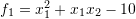and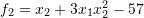. If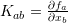, then it has the following form: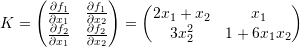Assuming an initial guess of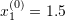and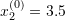, then the vector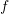and the matrixhave components: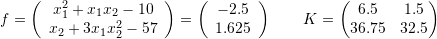The components of the vectorcan be computed as follows: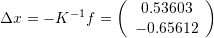Therefore, the new estimates for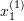and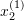are: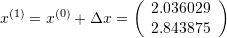The approximate relative error is given by: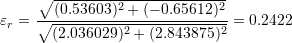For the second iteration the vectorand the matrixhave components: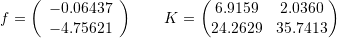The components of the vectorcan be computed as follows: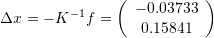Therefore, the new estimates for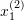and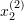are: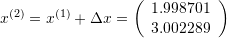The approximate relative error is given by: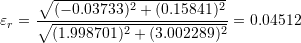For the third iteration the vectorand the matrixhave components: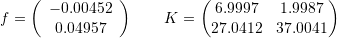The components of the vectorcan be computed as follows: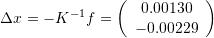Therefore, the new estimates for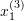and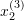are: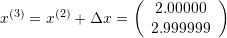The approximate relative error is given by: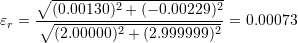Finally, for the fourth iteration the vectorand the matrixhave components: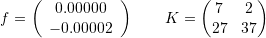The components of the vectorcan be computed as follows: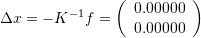Therefore, the new estimates for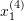and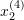are: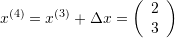The approximate relative error is given by: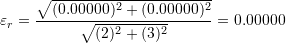Therefore, convergence is achieved after 4 iterations which is much faster than the 9 iterations in the fixed-point iteration method. The following is the Microsoft Excel table showing the values generated in every iteration: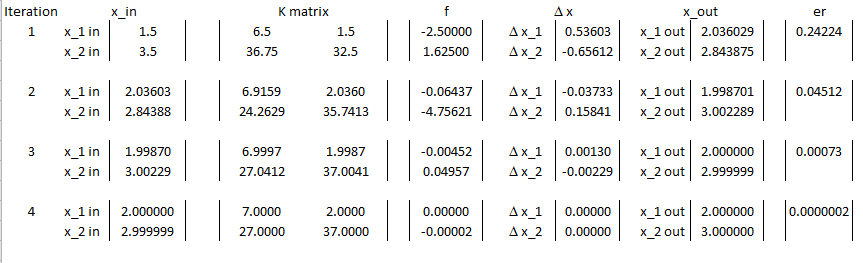The Newton-Raphson method can be implemented directly in Mathematica using the “FindRoot” built-in function:

View Mathematica Code
FindRoot[{x1^2 + x1*x2 - 10 == 0, x2 + 3*x1*x2^2 == 57}, {{x1, 1.5}, {x2, 3.5}}]

View Python Code
# Using Sympy
import sympy as sp
x1, x2 = sp.symbols('x1 x2')
sol = sp.nsolve((x1**2 + x1*x2 - 10, x2 + 3*x1*x2**2 - 57), (x1,x2), (1.5,3.5))
print("Sympy: ",sol)

# Using Scipy's root
from scipy.optimize import root
def equations(x):
x1, x2 = x
return [x1**2 + x1*x2 - 10,
x2 + 3*x1*x2**2 - 57]
sol = root(equations, [1.5,3.5])
print("Scipy: ",sol.x)


MATLAB code for the Newton-Raphson example
MATLAB file 1 (nrsystem.m)
MATLAB file 2 (f.m)
MATLAB file 3 (K.m)

The following “While” loop in Mathematica provides an iterative procedure that implements the Newton-Raphson Method in Mathematica and produces the table shown:

View Mathematica Code
x = {x1, x2};
f1 = x1^2 + x1*x2 - 10;
f2 = x2 + 3 x1*x2^2 - 57;
f = {f1, f2};
K = Table[D[f[[i]], x[[j]]], {i, 1, 2}, {j, 1, 2}];
K // MatrixForm
xtable = {{1.5, 3.5}};
xrule = {{x1 -> xtable[[1, 1]], x2 -> xtable[[1, 2]]}};
Ertable = {1}
NMax = 100;
i = 1;
eps = 0.000001

While[And[Ertable[[i]] > eps, i <= NMax],
Delta = -(Inverse[K].f) /. xrule[[i]];
xtable = Append[xtable, Delta + xtable[[Length[xtable]]]];
xrule = Append[
xrule, {x1 -> xtable[[Length[xtable], 1]],
x2 -> xtable[[Length[xtable], 2]]}];
er = Norm[Delta]/Norm[xtable[[i+1]]]; Ertable = Append[Ertable, er]; i++]
Title = {"Iteration", "x1_in", "x2_in", "x1_out", "x2_out", "er"};
T2 = Table[{i, xtable[[i, 1]], xtable[[i, 2]], xtable[[i + 1, 1]], xtable[[i + 1, 2]], Ertable[[i + 1]]}, {i, 1, Length[xtable] - 1}];
T2 = Prepend[T2, Title];
T2 // MatrixForm

View Python Code
import numpy as np
import sympy as sp
import pandas as pd

x1, x2 = sp.symbols('x1 x2')
x = [x1, x2]
f1 = x1**2 + x1*x2 - 10
f2 = x2 + 3*x1*x2**2 - 57
f = sp.Matrix([f1, f2])
K = sp.Matrix([[f[i].diff(x[j]) for j in range(2)] for i in range(2)])
xtable = [[1.5, 3.5]]
Ertable = 
NMax = 100
i = 0
eps = 0.000001
Delta = -(K.inv()*f)

while Ertable[i] > eps and i <= NMax:
er = np.linalg.norm(np.array(Delta.subs({x1:xtable[-2], x2:xtable[-2]})).astype(np.float64))/np.linalg.norm(np.array(xtable[i+1]).astype(np.float64))
Ertable.append(er)
i+=1

T2 = [[i + 1, xtable[i], xtable[i], xtable[i + 1], xtable[i + 1], Ertable[i + 1]] for i in range(len(xtable) - 1)]
T2 = pd.DataFrame(T2, columns=["Iteration", "x1_in", "x2_in", "x1_out", "x2_out", "er"])
display(T2)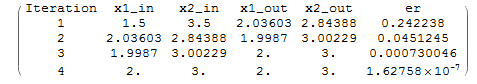### Lecture Video

1.Mohamed Naim Anwar says:
2.DUNCAN OTIENO OGANGA says: